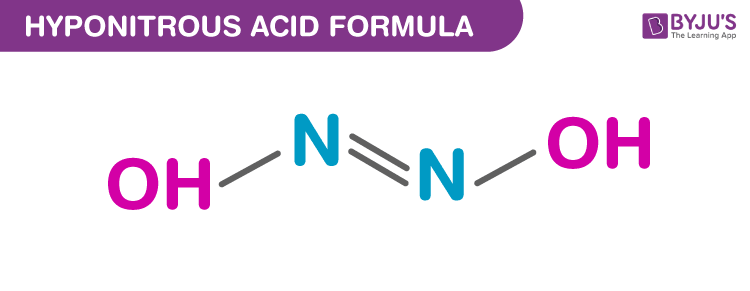# Hyponitrous Acid Formula

The hyponitrous acid is a chemical compound and an isomer of nitramide. The property value of hydrogen bond donor and hydrogen bond acceptor are 2 and 4 respectively. It is also known as a hyponitrous acid dimer.

There are two series of salts for hyponitrous acid and they are hyponitrites with [ON=NO]2− anion and acid hyponitrites with [HON=NO]. The property value of hydrogen bond donor and hydrogen bond acceptor is 2 and 4 respectively. Hyponitrous acid is synthesised from silver (I) hyponitrite and anhydrous HCl in the ether.

Following is the table of formulas of hyponitrous acid:

 Molecular formula H2N2O2 Linear formula HON=NOH Simplified molecular-input line-entry system (SMILES) N(=NO)O

## Structure Of Hyponitrous Acid

Trans and cis are the two possible structures of hyponitrous acid. White crystals are obtained from trans-hyponitrous which are explosive when dry and it is a weak acid which decomposes to nitrous oxide when in aqueous solution. Sodium salt can be obtained from cis acid.## Properties Of Hyponitrous Acid

 IUPAC name (E)-dihydroxydiazene Chemical formula H2N2O2 or HON=NOH Molecular weight 62.028 g/mol Conjugate base Hyponitrite

### Safety Measure

• It is an explosive in the dry form.